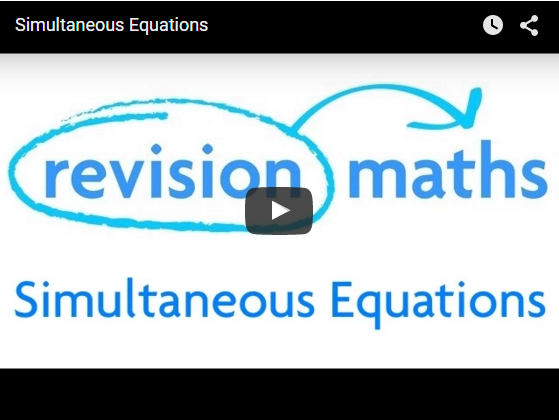# Hammond Maths- Simultaneous equations(revision world)

?

## Simultaneous Equations

Simultaneous equations and linear equations, after studying this section, you will be able to:

• solve simultaneous linear equations by substitution
• solve simultaneous linear equations by elimination
• solve simultaneous linear equations using straight line graphs

If an equation has two unknowns, such as 2y + x = 20, it cannot have unique solutions. Two unknowns require two equations which are solved at the sametime (simultaneously) − but even then two equations involving two unknowns do not always give unique solutions.

The video below works through examples of simultaneous equations. The step-by-step example shows how to group like terms and then add or subtract to remove one of the unknowns, to leave one unknown to be solved.This method is called solution by substitution.

It involves what it says − substitution − using one of the equations to get an expression of the form ‘y = …’ or ‘x = …’ and substituting this into the other equation. This gives an equation with just one unknown, which can be solved in the usual way. This value is then substituted in one or other of the original equations, giving an equation with one unknown.

NOTE:With this method you need to take particular care with your algebra. Should your solutions be ‘strange’ fractions such as 9/13 the chances are you’ve  made a slip − check your algebra.

Example

Solve the two simultaneous equations:

2y + x = 8 

1 + y = 2x 

from  y = 2x -1  ← subtract 1 from each side

Substituting this value for